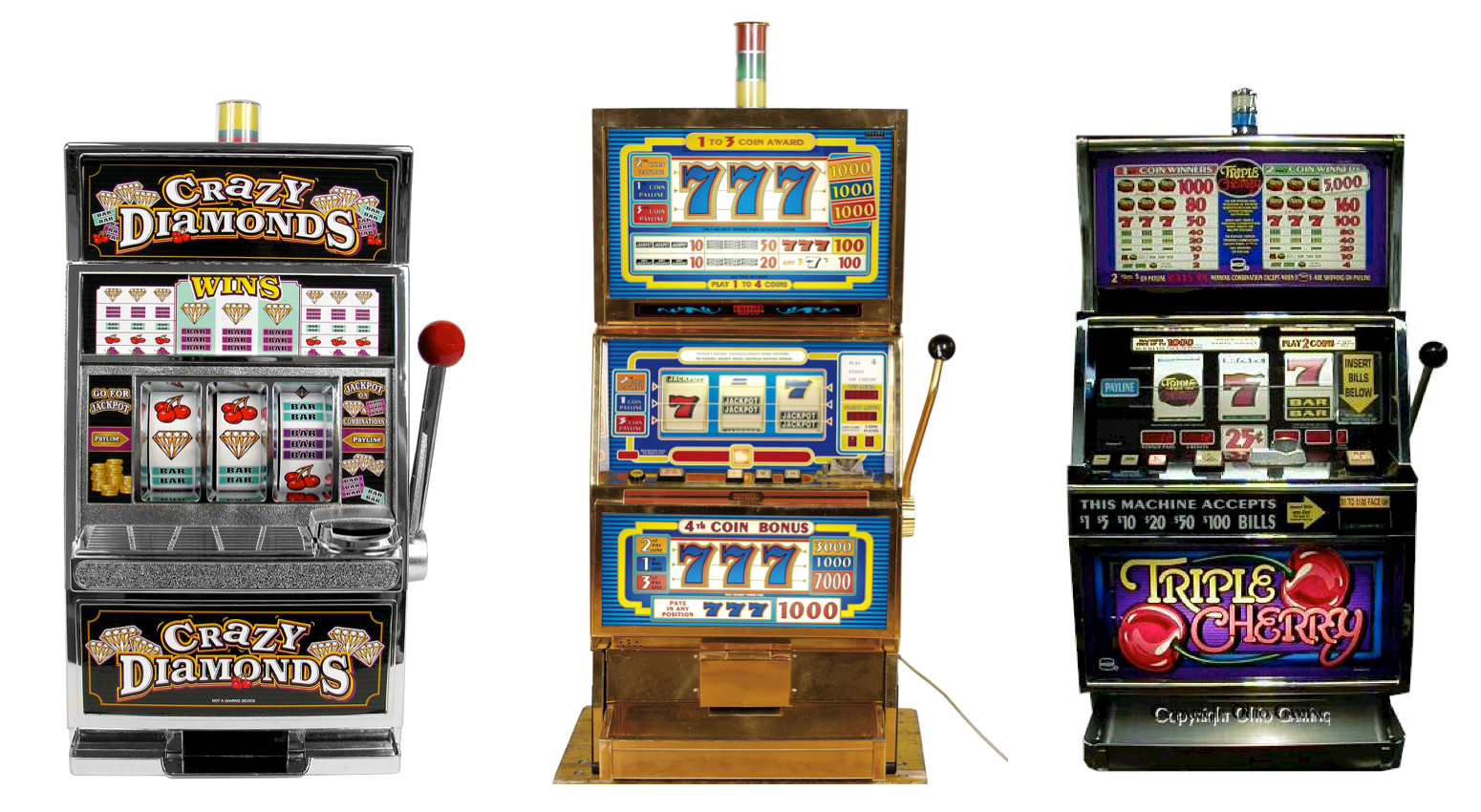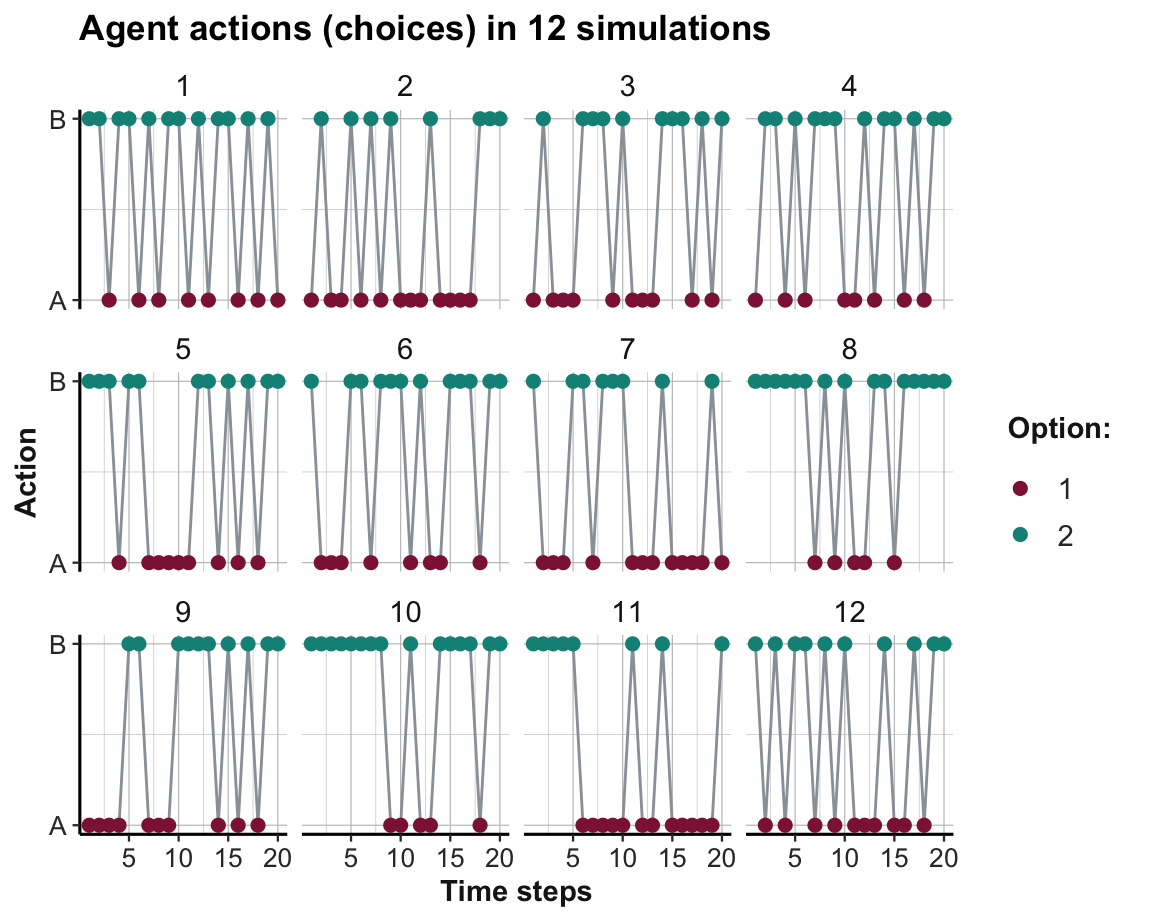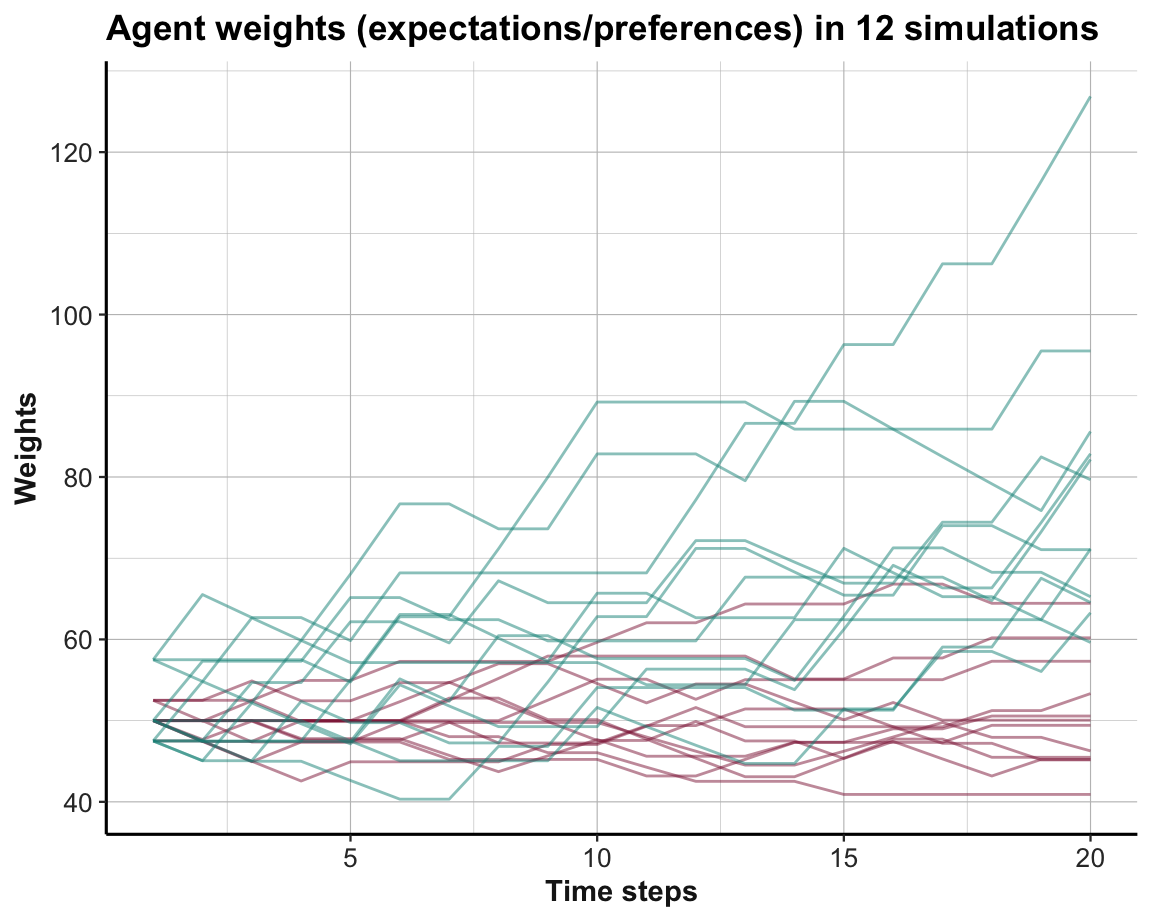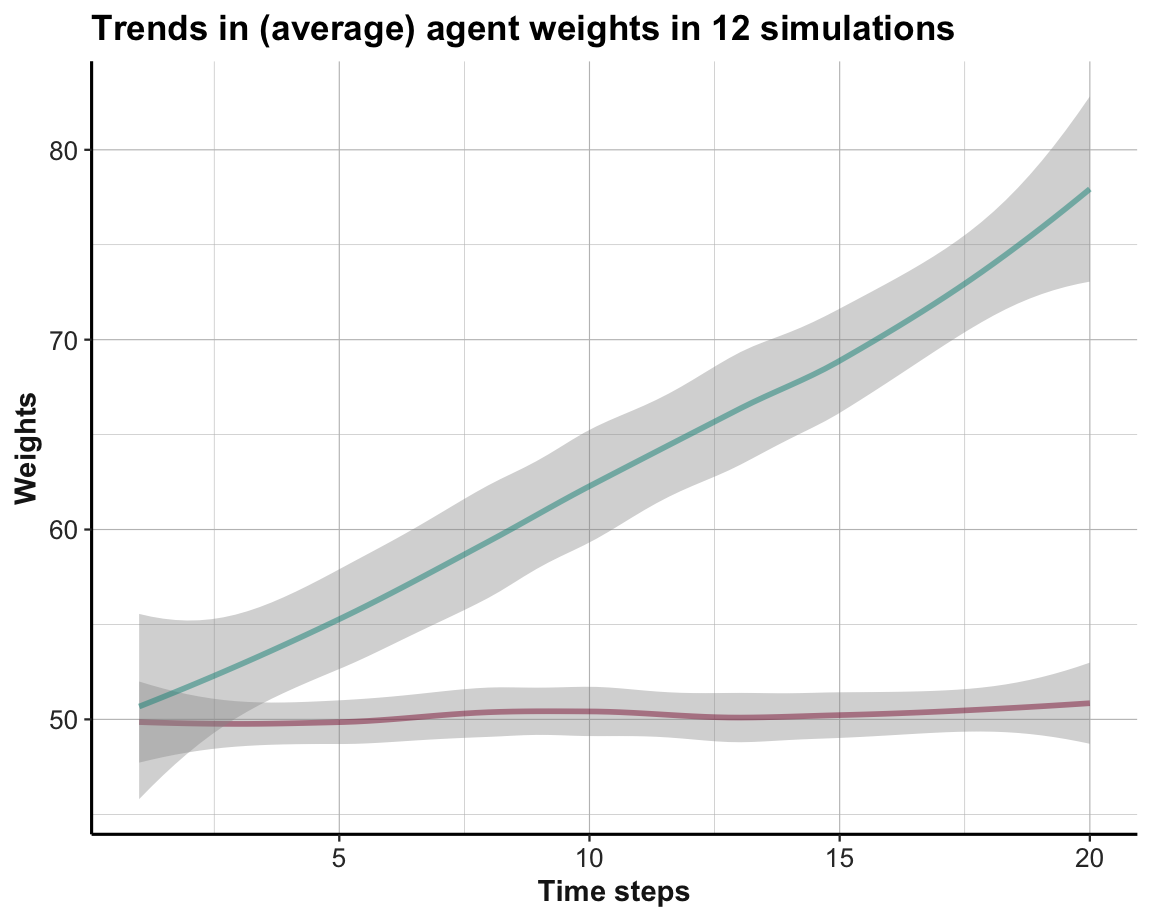## 16.3 Dynamic environments

Our discussion so far allowed for some flexibility in an agent. Evidence for adaptive adjustments in the agent’s internal state or behavior surfaced as evidence for learning, but the environment was assumed to be stable. In reality, however, environments are rarely stable. Instead, a variety of possible changes keep presenting new challenges to a learning agent.

### 16.3.1 Multi-armed bandits (MABs)

A seemingly simple and subtle step away from a stable environment consists in adding uncertainty to the rewards received from choosing options. Adding uncertainty to the rewards of options can be achieved by rendering their rewards probabilistic (aka. stochastic). This creates a large and important family of models that are collectively known as multi-armed bandit (MAB) problems. The term “bandit” refers to a slot machine in a casino that allows players to continuously spend and occasionally win large amounts of money. As these bandits typically have only one lever, the term “multi-arm” refers to the fact that we can choose between several options (see Figure 16.5).Figure 16.5: Three slot machines create a 3-armed bandit with uncertain payoffs for each option.

As all options are initially unfamiliar and may have different properties, an agent must first explore an option to estimate its potential rewards. With increasing experience, an attentive learner may notice differences between options and develop corresponding preferences. As soon as one option is perceived to be better than another, the agent must decide whether to keep exploring alternatives or to exploit the option that currently appears to be the best. Thus, MAB problems require a characteristic trade-off between exploration (i.e., searching for the best option) and exploitation (i.e., choosing the option that appears to be the best). The trade-off occurs because the total number of games (steps or trials) is finite (e.g., due to limitations of money or time). Thus, any trial spent on exploring an inferior option is costly, as it incurs a foregone payoff and reduces the total sum of rewards. Avoiding foregone payoffs creates a pressure towards exploiting seemingly superior options. However, we can easily imagine situations in which a better option is overlooked — either because the agent has not yet experienced or recognized its true potential or because an option has improved. Thus, as long as there remains some uncertainty about the current setup, the conflict between exploration and exploitation remains and must be negotiated.

MAB problems are widely used in biology, economics, engineering, psychology, and machine learning (see Hills et al., 2015, for a review). The main reason for this is that a large number of real-world problems can be mapped to the MAB template. For instance, many situations that involve a repeated choice between options (e.g., products, leisure activities, or even partners) or strategies (e.g., for advertising, developing, or selling stuff) can be modeled as MABs. Whereas the application contents and the mechanisms that govern the payoff distributions differ between tasks, the basic structure of a dynamic agent repeatedly interacting with a dynamic environment is similar in a large variety of situations. Thus, MAB problems provide an abstract modeling framework that accommodates many different tasks and domains.

Despite many parallels, different scientific disciplines still address different questions within the MAB framework. A basic difference consists in the distinction between theoretical vs. empirical approaches. As MABs offer a high level of flexibility, while still being analytically tractable, researchers in applied statistics, economics, operation research and decision sciences typically evaluate the performance of specific algorithms and aim for formal proofs of their optimality or boundary conditions. By contrast, researchers from biology, behavioral economics, and psychology are typically less concerned with optimality, and primarily interested in empirical data that informs about the strategies and rates of success when humans and other animals face environments that can be modeled as MABs. As both approaches are informative and not mutually exclusive, researchers typically need to balance the formal rigor and empirical relevance of their analysis — which can be described as a scientist facing a 2-armed bandit problem.

In the following, we extend our basic learning model by adding a MAB environment with probabilistic rewards. Chapter 2 of Reinforcement Learning: An Introduction by provides a more comprehensive introduction to MABs and corresponding models.

#### Basic idea

Assume that an agent is facing a choice between $$N$$ options that yield rewards $$\pi(1) ... \pi(N)$$ with some probability. In its simplest form, each option either yields a fixed reward (1) or no reward (0), but the probability of receiving a reward from each option is based on an unknown probability distribution $$p(1) ... p(N)$$. More generally, an option $$k$$ can yield a range of possible reward values $$\pi(k)$$ that are given by a corresponding probability distribution $$p(k)$$. As the rewards from such options can be analytically modeled by Bernoulli distributions, MAB problems with these properties are also called Bernoulli bandits (e.g., Page, 2018, p. 320). However, many other reward distributions and mechanisms for MABs are possible.

An agent’s goal in a MAB problem is typically to earn as much rewards as possible (i.e., maximize rewards). But as we mentioned above, maximizing rewards requires balancing the two conflicting goals of exploring options to learn more about them vs. exploiting known options for as much as possible. As exploring options can yield benefits (e.g., due to discovering superior options) but also incurs costs (e.g., due to sampling inferior options), the agent constantly faces a trade-off between exploration and exploitation.

Note that — from the perspective of a modeler — Bernoulli bandits provide a situation under risk (i.e., known options, outcomes, and probabilities). However, from the perspective of the agent, the same environment presents a problem of uncertainty (i.e., unknown options, outcomes, or probabilities).

#### Coding a model

To create a first MAB model, we extend the stable environment from above to a stochastic one, in which each option yields certain reward values with given probabilities:

# Initial setup (see Page, 2018, p. 308):

# Environment:
alt <- c("A", "B")  # constant options
rew_val <- list(c(10,  0), c(20,  0))  # reward values (by option)
rew_prb <- list(c(.5, .5), c(.5, .5))  # reward probabilities (by option)

# Agent:
alpha <- 1          # learning rate
A <- 5              # aspiration level
wgt <- c(50, 50)    # initial weights 

In the current model, we still face a binary-forced choice between the two environmental options given by alt, but now their rewards vary between some fixed value and zero (10 or 0 vs. 20 or 0, respectively) that occur with at a given rate or probability (here: 50:50 for both options). Note that both rew_val and rew_prb are defined as lists, as every element of them is a numeric vector (and remember that the i-th element of a list l is obtained by l[[i]]).

Recycling our learning agent from above, we only need to change the r() function that governs how the environment dispenses rewards:

# 1. Choosing:
p <- function(k){  # Probability of choosing k:

wgt[k]/sum(wgt)  # wgt denotes current weights

}

# Reward from choosing k:
r <- function(k){

reward <- NA

reward <- sample(x = rew_val[[k]], size = 1, prob = rew_prb[[k]])

# print(reward)  # 4debugging

return(reward)

}

# # Check: Choose each option N times
# N <- 1000
# v_A <- rep(NA, N)
# v_B <- rep(NA, N)
# for (i in 1:N){
#   v_A[i] <- r(1)
#   v_B[i] <- r(2)
# }
# table(v_A)
# table(v_B)

# 2. Learning:
delta_w <- function(k){ # Adjusting the weight of k:

(alpha * p(k) * (r(k) - A))

}

The functions for choosing options with probability p() and for adjusting the weight increment delta_w(k) of the chosen option k were copied from above. By contrast, the function r(k) was adjusted to now determine the reward of alternative k by sampling from its possible values rew_val[k] with the probabilities given by rew_prb[k].

Before running the simulation, let’s ask ourselves some simple questions:

• What should be learned in this setting?

• What aspects of the learning process change due to the introduction of stochastic options?

• Given the current changes to the environment, has the learning task become easier or more difficult than before?

We can answer these questions by copying the simulation code from above (i.e., only changing the environmental definitions in rew_val and rew_prb and their use in the r() function). Running this simulation yields the following results:

# Simulation:
n_S <- 12  # Number of simulations
n_T <- 20  # Number of time steps/cycles/rounds/trials (per simulation)

# Environment:
alt <- c("A", "B")  # constant options
rew_val <- list(c(10,  0), c(20,  0))  # reward values (by option)
rew_prb <- list(c(.5, .5), c(.5, .5))  # reward probabilities (by option)

# Prepare data structure for storing results:
data <- as.data.frame(matrix(ncol = (3 + length(alt)), nrow = n_S * n_T))
names(data) <- c("s", "t", "act", paste0("w_", alt))

for (s in 1:n_S){     # each simulation: ----

# Initialize agent:
alpha <- 1        # learning rate
A <- 5            # aspiration level
wgt <- c(50, 50)  # initial weights

for (t in 1:n_T){   # each step/trial: ----

# (1) Use wgt to determine current action:
cur_prob <- c(p(1), p(2))
cur_act <- sample(alt, size = 1, prob = cur_prob)
ix_act <- which(cur_act == alt)

# (2) Update wgt (based on reward):
new_w <- wgt[ix_act] + delta_w(ix_act)  # increment weight
wgt[ix_act] <- new_w  # update wgt

# (+) Record results:
data[((s-1) * n_T) + t, ] <- c(s, t, ix_act, wgt)

} # for t:n_T end.

print(paste0("s = ", s, ": Ran ", n_T, " steps, wgt = ",
paste(round(wgt, 0), collapse = ":")))

} # for i:n_S end.
#>  "s = 1: Ran 20 steps, wgt = 53:71"
#>  "s = 2: Ran 20 steps, wgt = 50:82"
#>  "s = 3: Ran 20 steps, wgt = 45:65"
#>  "s = 4: Ran 20 steps, wgt = 64:80"
#>  "s = 5: Ran 20 steps, wgt = 51:127"
#>  "s = 6: Ran 20 steps, wgt = 49:60"
#>  "s = 7: Ran 20 steps, wgt = 46:96"
#>  "s = 8: Ran 20 steps, wgt = 41:86"
#>  "s = 9: Ran 20 steps, wgt = 60:63"
#>  "s = 10: Ran 20 steps, wgt = 57:83"
#>  "s = 11: Ran 20 steps, wgt = 45:71"
#>  "s = 12: Ran 20 steps, wgt = 45:65"

# Report result:
print(paste0("Finished running ", n_S, " simulations (see 'data' for results)"))
#>  "Finished running 12 simulations (see 'data' for results)"

The feedback from running the model suggests that the model ran successfully and led to some changes in the agents’ wgt values. More detailed information on the process of learning can be obtained by examining the collected data (see below).

Before we examine the results further, note a constraint in all our implementations so far: As we modeled the reward mechanism as a function r() that is only called when updating the agent weights (in delta_w()), we cannot easily collect the reward values obtained in every round when filling data. If we needed the reward values (e.g., for adjusting the aspiration level in Exercise 16.6.1), we could either collect them within the r() function or change the inner loop (and possibly re-write the function delta_w()) so that the current reward value is explicitly represented prior to using it for updating the agent’s expectations (i.e., wgt).

Leaving some information implicit in a model is not necessarily a bug, as it may enable short and elegant models. However, a model’s level of abstraction crucially depends on how its functions are written — and we often need to compromise between formal elegance and practical concerns.

#### Visualizing results

As before, we can visualize the learning process and progress recorded in data. Figure 16.6 shows which option was chosen in each time step and simulation:

# Visualize results:
ggplot(data, aes(x = t)) +
facet_wrap(~s) +
geom_path(aes(y = act), col = Grau) +
geom_point(aes(y = act, col = factor(act)), size = 2) +
scale_color_manual(values = usecol(c(Bordeaux, Seegruen))) +
scale_y_continuous(breaks = 1:2, labels = alt) +
labs(title = paste0("Agent actions (choices) in ", n_S, " simulations"),
x = "Time steps", y = "Action", color = "Option:") +
theme_ds4psy()Figure 16.6: The agent’s action (i.e., option chosen) in a binary stochastic MAB per time step and simulation.

As before, Option B still yields higher rewards on average than Option A. However, due to the 50% chance of not receiving a reward for either option, the learning process is made more difficult. Although Figure 16.6 suggests that some agents develop a slight preference for the superior Option B, there are no clear trends within 20 trials. Figure 16.7 shows the option weights per time step for all 12 simulations:

ggplot(data, aes(x = t, group = s)) +
geom_path(aes(y = w_A), size = .5, col = usecol(Bordeaux, alpha = .5)) +
geom_path(aes(y = w_B), size = .5, col = usecol(Seegruen, alpha = .5)) +
labs(title = paste0("Agent weights (expectations/preferences) in ", n_S, " simulations"),
x = "Time steps", y = "Weights") +
theme_ds4psy()Figure 16.7: Trends in option weights in a binary stochastic MAB per time step for all simulations.

Although there appears some general trend towards preferring the superior Option B (shown in green), the situation is messier than before. Interestingly, we occasionally see that the option weights can also decline (due to negative $$\Delta w$$ values, when an option performed below the aspiration level $$A$$).

To document that some systematic learning has occurred even in the stochastic MAB setting, Figure 16.8 shows the average trends in the option weights per time step for all 12 simulations:

ggplot(data, aes(x = t)) +
geom_smooth(aes(y = w_A), size = 1, col = usecol(Bordeaux, alpha = .5)) +
geom_smooth(aes(y = w_B), size = 1, col = usecol(Seegruen, alpha = .5)) +
labs(title = paste0("Trends in (average) agent weights in ", n_S, " simulations"),
x = "Time steps", y = "Weights", col = "Option:") +
theme_ds4psy()Figure 16.8: Average trends in option weights in a binary stochastic MAB per time step for all simulations.

#### Interpretation

• The visualization of agent actions (in Figure 16.6) in the MAB setting shows that only a few agents learn to prefer the superior Option B in the n_T = 20 trials.

• The visualization of agent weights (in Figure 16.7) illustrates that agents generally preferred the superior Option B (shown in green) in the second half (i.e., trials 10–20) of the simulation. However, weight values can both increase and decline and the entire situation is much noisier than before, i.e., the preferences are not clearly separated in individual simulations yet.

• However, averaging over the weights for both options (in Figure 16.8) shows that the preference for the better Option B is being learned, even if it does not manifest itself as clearly in the choice behavior yet.

Overall, switching from a stable (deterministic) environment to an uncertain (stochastic) environment rendered the learning task more difficult. But although individual agents may still exhibit some exploratory behavior after n_T = 20 trials, we see some evidence in the agents’ average belief (represented by the average weight values wgt over n_S = 12 agents) that they still learn to prefer the superior Option B over the inferior Option A.

#### Practice

Answering the following questions improves our understanding of our basic MAB simulation:

1. Describe what the learning agent “expects,” “observes,” and how it reacts, when it initially selects an option, based on whether this option yields its reward value or no reward value.

2. Play with the simulation parameters (n_S and n_T) or agent parameters (alpha) to show more robust evidence for successful learning.

3. How would the simulation results change when the agent’s initial weights (or expectations) were lowered from wgt <- c(50, 50) to wgt <- c(10, 10)? Why?

4. Change the simulation code so that the reward value obtained on every trial is stored as an additional variable in data.

5. Imagine a situation in which a first option yields a lower maximum reward value than a second option (i.e., $$\max \pi(A) < \max \pi(B)$$), but the lower option yields its maximum reward with a higher probability (i.e., $$p(\max \pi(A)) > p(\max \pi(B))$$). This conflict should allow for scenarios in which an agent learns to prefer Option $$A$$ over Option $$B$$, despite $$B$$’s higher maximum reward. Play with the environmental parameters to construct such scenarios.

Hint: Setting the reward probabilities to rew_prb <- list(c(.8, .2), c(.2, .8)) creates one of many such scenarios. Can we define a general condition that states which option should be preferred?

### References

Hills, T. T., Todd, P. M., Lazer, D., Redish, A. D., Couzin, I. D., Cognitive Search Research Group, & others. (2015). Exploration versus exploitation in space, mind, and society. Trends in Cognitive Sciences, 19(1), 46–54. https://doi.org/10.1016/j.tics.2014.10.004
Page, S. E. (2018). The model thinker: What you need to know to make data work for you. Basic Books.
Sutton, R. S., & Barto, A. G. (2018). Reinforcement learning: An introduction (2nd ed.). MIT press. http://incompleteideas.net/book/the-book.html